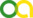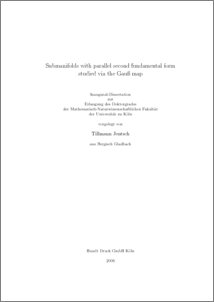Jentsch, Tillmann (2006). Submanifolds with parallel second fundamental form studied via the Gauß map. PhD thesis, Universität zu Köln.Preview
PDF
Diss.pdf

## Abstract

For an arbitrary n-dimensional riemannian manifold N and an integer m between 1 and n-1 a covariant derivative on the Graßmann bundle G_m(TN) is introduced which has the property that an m-dimensional submanifold M of N has parallel second fundamental form if and only if its Gauß map (defined on M with values in G_m(TN)) is affine. (For the case that N is the euclidian space this result was already obtained by J.Vilms in 1972.) By means of this relation a generalization of E. Cartan's theorem on the total geodesy of a geodesic umbrella can be derived: Suppose, initial data (p,W,b) prescribing an m-dimensional tangent space W and a second fundamental form b at p in N are given; for these data we construct an m-dimensional ``umbrella'' M=M(p,W,b) in N, the rays of which are helical arcs of N; moreover we present tensorial conditions (not involving the covariant derivative on G_m(TN)) which guarantee that the umbrella M has parallel second fundamental form. These conditions are as well necessary, and locally every submanifold with parallel second fundamental form can be obtained in this way.

Item Type: Thesis (PhD thesis)
Creators:
CreatorsEmailORCIDORCID Put Code
Jentsch, Tillmanntjentsch@math.uni-koeln.deUNSPECIFIEDUNSPECIFIED
URN: urn:nbn:de:hbz:38-17915
Date: 2006
Language: English
Faculty: Faculty of Mathematics and Natural Sciences
Divisions: Faculty of Mathematics and Natural Sciences > Department of Mathematics and Computer Science > Mathematical Institute
Subjects: Mathematics
Uncontrolled Keywords:
KeywordsLanguage
parallel submanifold , Graßmann bundle , Gauß mapEnglish
Date of oral exam: 11 July 2005
Referee:View Item